# Fundamental philosophy

In this chapter, we will explain the fundamental philosophy of definitive programming and the language Eden.

## Concept of Eden programming

The important ingredients of the Eden language are the formula definitions and action specifications. In this section, we explain these concepts.

### Formula Definition

A definition of an object is expressed as a mathematical expression. A variable is called a formula variable if its value is defined in terms of other variables, e.g: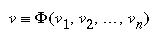where v is the name of the formula variable, and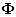represents an expression with the variables v1, v2, ... , vn involved. The variables v1, v2, ... , vn are called the source variables of this definition since the value of v is computed from their values. Hence the formula variable v, can also be called the target variable. Eden supports the concept of definition. A formula definition has the form: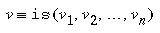The keyword ``is'' defines a formula variable v whose value is computed from the values of source variables v1, v2, ..., vn and the expression on the right hand side, denoted by, is the formula of the variable $v$. In other words, a formula describes how the value of a variable is computed from other data. These formulae are permanently valid (unless they are re-defined). That is, no matter what the values of source variables are, the value of variable $v$ is always equal to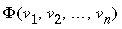. Thus a formula gives an abstract definition of a variable rather than the explicit value of it. This is the major difference between formula definitions and assignment statements. For example, after executing the assignmentv is equal toonly after the expressionis evaluated and before any of the values of the source variables is altered.

Unlike the assignment statement, the formula definition, itself, is not an executable statement. When and how the formula expression defined in the definition is managed by the system. However, the values of the formula variables are guaranteed to be up-to-date when the variables are examined.

Notice that a definition does not imply the inverse definitions (where these exist); for example, the definition (2-1) does not imply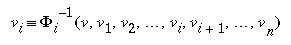where 1<=i<=n

A definition can be a representation of a real relationship among objects; for instance, ``a lamp is placed (somewhere) on a table'' can be formulated as:

```lamp_position is table_position + something
```

If the table is re-positioned the lamp will also be moved because the definition has specified the position of the lamp in terms of the position of the table, the system can automatically keep track of these definitions and re-calculate `lamp_position` whenever `table_position` has been changed. But if the `lamp_position` is then redefined to

```lamp_position is desk_position + something
```

we mean to put the lamp on the desk instead of the table. The table remains at the same position because it is not defined in terms of the lamp. Note that the new definition of `lamp_position` overwrites the previous definition. Hence the lamp can be moved independently by redefining its definition.

### Action Specification

So far, we have talked about formula definitions without mentioning the updating actions (e.g. renew the display of the content of a variable when it is changed) since there is no default implicit action associated with each definition. However, Eden provides a way of defining explicit actions. Thus the user can specify special updating actions for different devices. In the rest of this handbook, the term ``action'' refers to ``explicit action'' because it is the only kind of action available in Eden.

The following statement illustrates a sample action definition.

```proc display_v : v { display(v); }
```

The keyword ```proc`'' defines an action, named as `display_v`, which invoked by the system when the value of variable `v`, (specified after the colon) is changed. The curly brackets `{}` enclose a list of statements to be executed sequentially. In this case, there is only one procedure call. The procedure `display` is defined separately. By calling different functions (with appropriate side-effects, e.g. `writeln(...)` prints the values of its arguments on the standard output) in the function libraries, display can do different updating actions. This makes Eden more extensible.

Note that the value of `v` is not passed as a parameter to `display_v` when the action is invoked, but since it is a global variable, it can be accessed by the action directly.

## The EDEN system

The Eden language has definitions, actions and some conventional features. The conventional features are, for example, iterative loops, flow control statements, user-defined functions and read/write variables.

The variables can be classified into 4 types: read/write variables, functions, formula variables, and action specifications. Eden has different statements to assign values to these variables.

Read/write variables (RWV) are the same as the variables of conventional languages. As the name implied, the value of a RWV can be assigned and examined by the user. For instance,

```A = 1
```

will assign integer `1` to the RWV `A`. The value of a variable is referenced if the variable appears in an expression - e.g. the right-hand side of the assignment statement. For example,

```B = A
```

puts the value of `A` into RWV `B`.

Note that, in EDEN, a function is considered as a RWV (although there is a different instruction to define a function, and some restrictions on their definition). Functions will be discussed in the later chapters.

## Formula Variables

A formula variable (FV) consists of a data register (DR) and a formula expression (FE) (or simply formula). The formula describes how the value of the data register (DR) can be computed from other variables (RWV or FV). The system will always update the DR using the formula whenever it is possible. This part of the system is called formula data maintainer (FDM).

The value of a formula is equivalent to the value of the DR of that FV. The user can (re-)define the formula of a definition but not the value of the DR. This means that the DR is read-only by the user. In Eden, the formula of a formula variable is defined using the ```is`'' operator, for example:

```f is a + b
```

defines a FV `f` whose value is always equal to the sum of the values of variables `a` and `b`. The expression ```a + b`'' is the FE of `f`. Note that all variables mentioned in the FE, in this case, `a` and `b`, can be either FV's or RWV's.

The value of a FV is referenced if the name of the FV appears in an expression, e.g. the right-hand side of an assignment statement.

The syntax of a formula definition is defined in the Definitive statements section of this document.

## Block Diagram of a Formula Maintaining Sub-system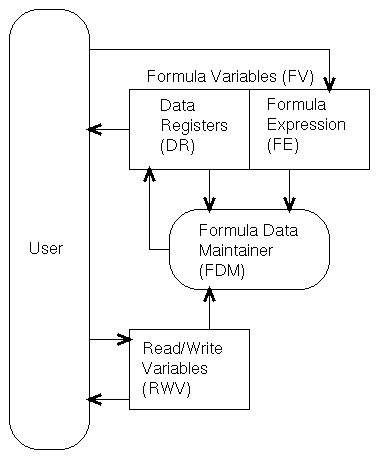The arrows show how data might flow. E.g. the user can define a FV by giving the formula to it. As shown in the diagram, the user can examine the value of a DR but not write to it. When the user (re)defines a FV or writes to a RWV, the FDM will re-calculate those DR's using their associated FE's. The FDM will read the values of some RWV's and/or DR's if it is necessary. The results will be assigned to the DR's by the FDM.

## Action Specification

An action specification (AS) is a named sequence of instructions. This sequence of instructions will be invoked by the system whenever the values of any source variables, specified explicitly in a list, are changed. The term ``changed'' is causally defined. It may mean the value of a variable is different from the previous one, or the value of a variable is overwritten (by the user or by the system) though the value may be the same as the previous value. EDEN takes the latter definition. This definition of ``changed'' is used throughout this handbook unless otherwise specified.

An example of an action definition is:

```proc print_sum : a, b, c { writeln("a+b+c=", a+b+c); }
```

This action, named as `print_sum`, will invoked by the system whenever the values of the source variables `a`, `b` or `c` (listed after the colon) are changed. The action will print a string and the sum of the values of these variables on the screen due to the side-effect of the pre-defined function `writeln`.

The action execution manager (AEM) is responsible for invoking the action. This sub-system is called the action execution management sub-system.

## Block Diagram of the Action Execution Management Sub-system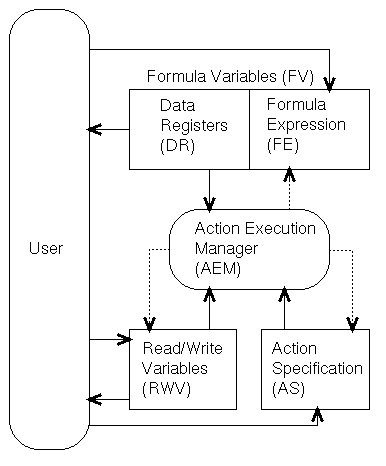The arrows indicate the possible data flow in the system. Shaded arrows indicate the data flow due to the side-effects of action execution. From this diagram, we see that the actions are invoked by the AEM. AEM loads the procedure of the appropriate action from the AS and then executes it. The execution may cause a write to a (or some) RWV, or (re)define FV's or AS's (using some tricks). Of course, the action may also access I/O devices (not shown in the diagram).

## Execution Strategy

Eden is neither purely declarative (in which case the execution strategy does not affect the result) nor purely procedural (some side-effect are triggered rather than called explicitly). Therefore, the user needs to aware of the execution strategy in order to make the most out of Eden.

EDEN takes precedence of formula evaluation over actions. This means that EDEN will update all the value changes to the variables first. The triggered actions are queued in an action queue. After all the variables are updated, those actions queued will be performed. This will ensure that any reference to a variable will get the most up-to-date value.

EDEN also uses a breath-first scheme of evaluation. Under such scheme, the interpreter spends more time on scheduling the order of evaluation than a depth-first scheme but it has an advantage of doing the evaluation of variable or the triggered actions only once in each phase. For example:

```proc dummy : A {  /* dummy() will be executed when A changes */
auto i;   /* local variable */
for (i = 1; i <= 10; i++) {
V = i;
}
}

proc Print_V : V {
write(V, ' ');
}

A = 1;     /* change A to trigger dummy() */
```

the interpreter will produces the output:

```        10
```

rather than

```        1 2 3 4 5 6 7 8 9 10
```

While the depth-first scheme is good at monitoring the change of values, the breath-first scheme the current implementation using is more efficient in producing the final result. Should the later output is preferred, the user has to force the interpreter to execute the actions in the action queue by a eager() statement. For the above example, the required result can be generated if dummy is alternatively defined as:

```proc dummy : A {   /* dummy() will be executed when A changes */
auto i;   /* local variable */
for (i = 1; i <= 10; i++) {
V = i;
eager();   /* force execution of action queue */
}
}
```

One should not presume the order of action execution. A general principle is that if the order of execution will significantly affect the result, it is likely that it is not a good program. However, some sort of ordering is necessary. A typical example is the simulation of a clocked system. In a clocked system, there should be actions (NB the clocking mechanism cannot be replaced by a for/while loop, because within a loop the EDEN interpreter will not accept any user input, and the user cannot interact with the simulation) such as:

```proc clocking : clock {   /* a clocking process */
clock++;           /* advance the clock */
}

proc device1: clock {  /* action synchronised by clock */
...   /* action body */
}

proc device2: clock {
...   /* action body */
}

...
```

In these cases, the `clocking` action should be executed last so that it would not block out other actions to be performed in this clock cycle. To solve this scheduling problem, EDEN has a to-do list (in fact two to-do lists, one currently executing and the other one is really to be executed). The clocking action above should be rewritten as:

```proc clocking : clock {   /* a clocking process */
todo("clock++;");        /* advance the clock in future */
}
```

This will cause the statement:

```clock++;
```

to be saved in the (future) to-do list. After all the triggerred actions and things-to-do in the current to-do list have been executed, the system will perform those statements in the future (now becomes the current) to-do list. In this case, when `clock++` is executed, all other actions will be triggered and executed in this 'clock cycle' and another `clock++` statement is scheduled to do after all the necessary actions are performed in this clock cycle.